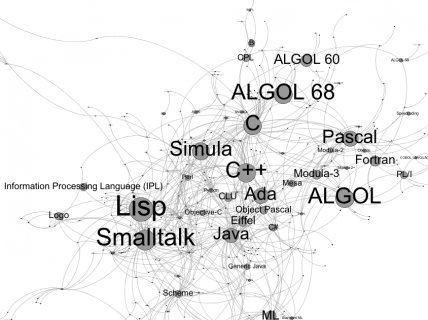Examples semantics programming languages

The Semantics of Programming Languages

Semantics, roughly, are meanings given for groups of symbols: ab+c, "ab"+"c", mult(5, 4).

For example, to express the syntax of adding 5 with 4, we can say: Put a "+" sign in between the 5 and 4, yielding "5 + 4". However, we must also define the semantics of 5+4. If we use arithmetic semantics, 5+4 would be a representation/equivalent(=) of 9.

In computer science, the author of a programming language 'could' either use or create a new definition for '+'. For example, '+' can defined as arithmetic subtraction(-). More commonly however, '+' is defined as a function(takes in a value to output another) for complex numbers, and even strings(an ordered set of symbols) like "123a", "aFsd", and "./etc".

Semantics are from being mere theory or philosophy. Many properties of programming languages can only be determined through rigorous analysis. For example, we'd like to make statements such as "this programming language is secure." But to demonstrate that a programming language is secure requires more than just a discussion of the language's syntax. Without a formal proof of security, systems can be vulnerable to the unanticipated consequences of many separate interacting concerns. One way to make such proofs is by using mathematical models.

Given the semantics of one programming language we have the option of describing other programming languages in terms of that base language. So, one way to specify the semantics of a programming language is to relate it to another language. However, this creates a bootstrapping problem: what to use for the original base language. Programming language researchers have solved the problem by using a mathematical model that was originally invented for logic, but has dramatic computational applications: Lambda Calculus.

Lambda Calculus, invented in the 1920s and 30s by Church, Kleene, and others, is one of the simplest to describe Turing Complete languages. It's a language that only has a single value: the function; and only two operations: defining a function and calling a function. To make things even simpler, functions are only allowed to have one parameter.

In the following discussion, gear your mind to thinking about functions that take functions as arguments and return other functions as values. It is a very abstract concept, but, as we'll find, soon we can build up the language through clever definitions into something that looks more like the programming languages we are used to. For example, one of the first tricks we'll explore is how to achieve the same result of allowing multiple parameters even though each function can only take a single parameter.

 An etymological dictionary or analysis of the English language: containing the radicals and definitions of words derived from the Greek, Latin, and French ... and all the generaly used technical eBooks